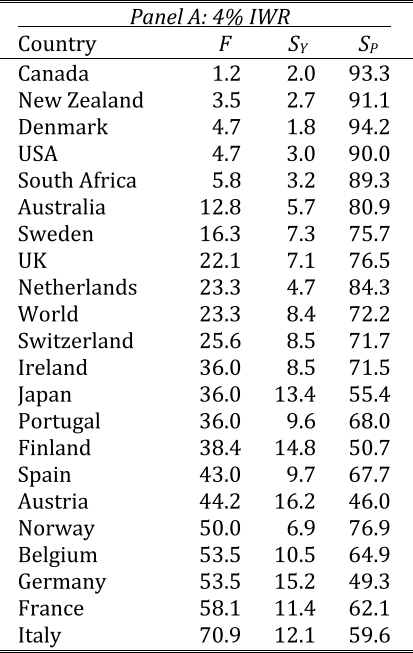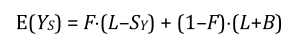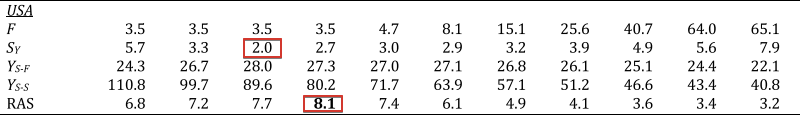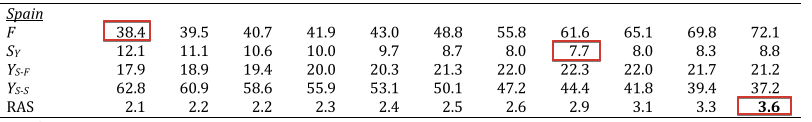# Shortfall Years

A common complaint about failure rates is that “it assumes investors are indifferent between running out of money early in retirement or near the end of retirement”. That is, running out of money in Year 17 is worse than running out of money in Year 29 (when you are almost certainly dead anyway). But they are treated identically by failure rate metrics.F is the failure rate. Sy is the average number of shortfall years when failure did occur. Sp is the percentage of the retirement sustained, on average.

# Risk-Adjusted Success (RAS)

The next was a paper the following year, “From Failure to Success: Replacing the Failure Rate”, which looked at shortfall years across a variety of asset allocations and introduced the RAS metric.

## RAS: Years Sustained

First we want to calculate the “years sustained”. When a portfolio fails, the number is easy. We just subtract the shortfall years from the target length of retirement. If our target was 30 years and we had a 3 year shortfall then “years sustained” is 27.

## RAS: Expected Value

We can put together all of “years sustained” to form an “expected value”. Expected value is just a calculation that takes probability into account. If I have a 10% chance of winning $100 and a 90% chance of losing -$50 then my expected value is (.10 x 100) + (.90 x -50) = (10) + (-45) = -35.F is the failure rate. L is the fixed retirement length (i.e. 30). Sy is the average number of shortfall years. B is the average number of “extra years”.
1. Calculate how long the portfolio lasts (on average) when it fails. e.g. the average shortfall is 3.7 years. So the average “years sustained” when the portfolio fails is 26.3 years.
2. Then we multiply that by the failure rate. (e.g. continued) The failure rate is 3.5%. 0.035 x 26.3 = 0.9205.
3. Calculate how long the portfolio lasts (on average) when it succeeds. (e.g. continued) We look at the final portfolio size and find that, on average, it resulted in 6.7 extra years. So the “years sustained” is 36.7.
4. Then we multiply that by the success rate. (e.g. continued) The success rate is 96.5%. 0.965 x 36.7 = 35.4155.
5. Then we add the two numbers together. 0.9205 + 35.4155 = 36.336.

## RAS: standard deviation of years sustained

The next step is calculate the standard deviation of all those years sustained. This is the volatility of how long the portfolio lasts.

=(0.3333 * 27) * (0.6666 * 33.5)=31.3333
a = (0.3333 * (27 - 31.3333))b = (0.6666 * (33.5 - 31.3333))= sqrt((a*a) + (b*b))= 3.064

## RAS: Putting it all together

The standard deviation along only tells us about volatility, not about how long the portfolio lasts. You could have a strategy that always lasts exactly 26 years. Low volatility but also low expected value.

=31.333 / 3.064=10.226
1. The expected value is high. That is, on average, the portfolio lasts longer.
2. The standard deviation is low. That is, there is more certainty that we’ll end up close to the expected value.
1. It treats upside & downside as symmetric. But investors clearly don’t think that way. No one says “my retirement was a failure, my portfolio ended up twice as big as I expected”.
2. A portfolio with very low volatility is going have a very high RAS, regardless of how low the expected value is. Our example above, of the strategy that always lasts exactly 26 years has an amazingly good RAS, infinite in fact, even though few people would want to risk using a strategy with such a low expected value.Each column is a different asset allocation. 100/0 on the far left down to 0/100 on the far right.Each column is a different asset allocation. 100/0 on the far left down to 0/100 on the far right.

# Downside Risk-Adjusted Success (D-RAS)

Another paper the same year, “Replacing the Failure Rate: A Downside Risk Perspective”, set out to address problem #1. We shouldn’t treat upside and downside volatility the same.

# Coverage Ratio

The next step came in the paper “Evaluating Retirement Strategies : A Utility-Based Approach” where Estrada teamed with Mark Kritzman for the first time. Their calculation is performed in two steps:

1. The coverage ratio is defined as the ratio of the “years sustained” and the target length of retirement. If a portfolio last 28 years out of 30 then its coverage ratio would be 28/30 = 0.9333. If a portfolio lasted 43 out of 30 then its coverage ratio would be 43/30 = 1.43333
2. Next apply a utility function. This discounts the value of outperformance and penalizes underperformance.
# a portfolio that lasts exactly 30 years, as planned.u(30 / 30) = 0.0# a portfolio that lasts 1 year extra only get a small bonusu(31 / 30) = 0.0327# the bonus DOESN'T increase linearly. u(40 / 30) = 0.2877# a portfolio that falls short by 1 year has a large penaltyu(29 / 30) = -0.3334# the penalty DOES continues to increase linearlyu(20 / 30) = -3.333

# “Toward Determining the Optimal Investment Strategy for Retirement”

Finally we turn to their most recent paper, which came out December 2018/early 2019.